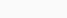# GRADE 6 – SAMPEL PAPER FINAL FMO 2021

Berikut ini adalah soal beserta kunci jawaban Fermat Mathematic Olympiad (FMO) 2021 grade 6 (Sc: Edukultur Indonesia)

A. Warm-up (4 points per question / No points deducted for wrong answers)

1. A rectangular piece of land is 27m long. The width is $$\frac{2}{3}$$ the length. They use $$\frac{5}{9}$$ of the land’s area to plant flowers, $$\frac{1}{6}$$ of the land’s area for the walking path. The rest is used to build a pond. Find the area of that pond.
A) 270 $$m^2$$
B) 135 $$m^2$$
C) 490 $$m^2$$
D) 810 $$m^2$$
E) 630 $$m^2$$

2. A digital clock shows the time right now which is 20:30. After 20:30, 4 digits reappear at 23:00 for the first time. How long will it be from now until those 4 digits appears for the second time?A) 150 min
B) 233 min
C) 242 min
D) 360 min
E) None of the above

3. The students at Webster Middle School are raising money for after-school programs. Which month did both grades raise the most money altogether?A) June
B) July
C) August
D) September
E) October

4. Gasoline costs 6 dollars per liter. A car uses up 8 liters for every 100 km.     What is the largest integral number of km that can be covered with 200 dollars worth of gasoline?
A) 416
B) 417
C) 418
D) 419
E) 420

5. Teacher has a square piece of paper. She folds it in half twice then cuts along the dotted line as the figure below. How many pieces does she get after cutting?A) 4
B) 5
C) 9
D) 12
E) 16

B. Speed-up (6 points per question / No points deducted for wrong answers)

6. Anna has 2 rectangular papers with area $$24cmˆ2$$ and $$48cmˆ2$$ respectively. She combines them with a square paper to get the figure below with total area $$108cmˆ2$$. Find the perimeter of the combined figure.A) 46cm
B) 52cm
C) 44cm
D) 56cm
E) 48cm

7. Each friend choose 2 distincts from these digits: 0, 1, 3, 5, 7, 9. No two friends choose the same digit. Mike’s number is a prime number. Bob’s number divisible by 6. John’s number is divisible by 25. What can be the greatest value of Mike’s number?
A) 13
B) 19
C) 91
D) 31
E) 59

8. A cat named Kitty is living in a 3-room house. Every morning, Kitty goes from the living room to the kitchen to eat, then goes outside. Finally she goes to the bedroom without going through the kitchen. Given that Kitty must enter the room through the given doors on the map, in how many different ways can she complete her routine?A) 4
B) 6
C) 8
D) 7
E) 5

9. The total of each row and column is given as below. What is the valueA) 13
B) 14
C) 15
D) 16
E) 17

10. How many small squares are there in the 50th figure?A) 150
B) 151
C) 152
D) 153
E) None of the above

C. Challenge (8 points per question / No points deducted for wrong answers)

11. In a basket, there are 30 fruits with apples, bananas and mangoes. Grandma exchange 6 apples for 3 bananas. Then she exchange 2 bananas for 8 mangoes. Now there is the same number of apples, bananas and mangoes in the basket. How many bananas were there in the beginning?
A) 4
B) 5
C) 11
D) 10
E) 9

12. Two cubes of side length 4dm and one cube of side length 8dm are put beside each other as in figure below. Then they paint all the surface of the shape (except for the bottom). Find the area of the painted region.A) $$400dmˆ2$$
B) $$512dmˆ2$$
C) $$596dmˆ2$$
D) $$416dmˆ2$$
E) $$384dmˆ2$$

13. While reading a book, Wendy reads one page more each day than the preceding day. After 4 days, she has read 150 pages. In exactly 10 days, she finishes the book. How many pages are there in this book?
A) 395
B) 405
C) 375
D) 410
E) 385

14. A man cycles to the park and goes back home. Then he walks to the coffee shop to buy some drink and walks back home again. His distance from home is shown in the chart below. How far is the park from his house given that his average speed in the whole trip is 12.6 km/h?A) 3km
B) 2km
C) 5km
D) 1km
E) 6km

15. A train is made up of 21 carriages. There are 500 passengers travelling on the train. In any block of five adjacent carriages, there are 120 passengers in total. How many passengers are there in the middle carriage?
A) 60
B) 50
C) 40
D) 30
E) 20

16. Two numbers in the $$4\times4$$ grid below can be swapped to create a Magic Square (in which the sum of every row, every column and every main diagonal is the same). What is the sum of these two numbers?